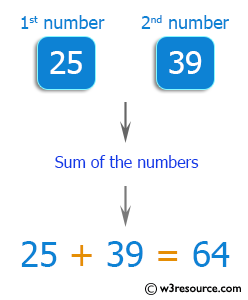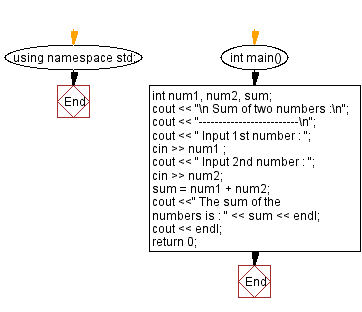﻿ C++ Exercises: Add two numbers accept through keyboard - w3resource

# C++ Exercises: Add two numbers accept through keyboard

## C++ Basic: Exercise-12 with Solution

Write a C++ program to add two numbers and accept them from the keyboard.

Pictorial Presentation:Sample Solution:

C++ Code :

``````#include <iostream>
using namespace std;

int main()
{
int num1, num2, sum;
cout << "\n Sum of two numbers :\n";
cout << "-------------------------\n";
cout << " Input 1st number : ";
cin >> num1 ;
cout << " Input 2nd number : ";
cin >> num2;
sum = num1 + num2;
cout <<" The sum of the numbers is : " << sum << endl;
cout << endl;
return 0;
}
``````

Sample Output:

``` Sum of two numbers :
-------------------------
Input 1st number : 25
Input 2nd number : 39
The sum of the numbers is : 64
```

Flowchart:C++ Code Editor:

What is the difficulty level of this exercise?

﻿

## C++ Programming: Tips of the Day

Why is there no std::stou?

The most pat answer would be that the C library has no corresponding "strtou", and the C++11 string functions are all just thinly veiled wrappers around the C library functions: The std::sto* functions mirror strto*, and the std::to_string functions use sprintf.

Ref: https://bit.ly/3wtz2qA

We are closing our Disqus commenting system for some maintenanace issues. You may write to us at reach[at]yahoo[dot]com or visit us at Facebook